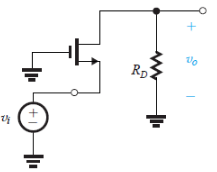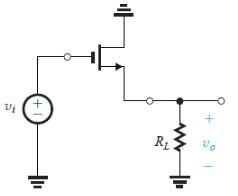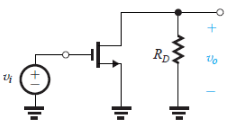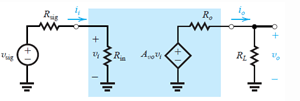# Test: Basic MOSFET Amplifier Configurations

## 10 Questions MCQ Test Analog Circuits | Test: Basic MOSFET Amplifier Configurations

Description
Attempt Test: Basic MOSFET Amplifier Configurations | 10 questions in 10 minutes | Mock test for Electronics and Communication Engineering (ECE) preparation | Free important questions MCQ to study Analog Circuits for Electronics and Communication Engineering (ECE) Exam | Download free PDF with solutions
QUESTION: 1

### In which of the following configuration does a MOSFET works as an amplifier?

Solution:

There are three basic configurations for connecting the MOSFET as an amplifier. Each of these configurations is obtained by connecting one of the three MOSFET terminals to ground, thus creating a two-port network with the grounded terminal being common to the input and output ports.

QUESTION: 2

### The MOSFET in the following circuit is in which configuration?​Solution:

It is the circuit for Common gate configuration.

QUESTION: 3

### The MOSFET in the following circuit is in which configuration?Solution:

It is the circuit for Common drain configuration.

QUESTION: 4

The MOSFET in the following circuit is in which configuration?[/expand]​Solution:

It is the circuit for Common source configuration.

QUESTION: 5

(Q.5-Q.10) Reference circuit for Q.5-Q.10 The circuit below is the characterization for the amplifier as a functional block.If the value of Rin for the common source configuration is R1 and that for common source with a source resistance configuration is R2 ideally. The ratio of R1/R2 will be

Solution:

Ideally both must have infinite resistance.

QUESTION: 6

Which is true for the value of Avo for common source (Represented by A1) and common source with a source resistance (represented by A2).

Solution:

A1 = -gmRD and A2 = -gmRD/1+gmRS
Reference circuit for Common source configuration

QUESTION: 7

Which of the following is true for the voltage gain (AV) for the common source configuration (represented by A1) and the common gate configuration (represented by A2)?

Solution:
QUESTION: 8

The value of the voltage gain (Av) for the common source with source resistance (represented by A1) and common gate configuration (represented by A2) are related to each other by

Solution:
QUESTION: 9

In which of the following configuration is the input resistance (Ri) not equal to zero ideally?

Solution:
QUESTION: 10

Which of the following has AVO independent of the circuit elements?

Solution:

AVO = 1 source follower.Use Code STAYHOME200 and get INR 200 additional OFF Use Coupon Code